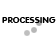Ambient Conditions:
The conditions (humidity, pressure, temperature, etc.) of the medium surrounding the load cell.
Ambient Temperature:
The temperature of the medium surrounding the load cell.
A load applied eccentric with the primary axis at the point of application and at some angle with respect to the primary axis.
A load applied concentric with the Primary axis at the point of application and at some angle with respect to the Primary axis.
A load applied along or parallel to and concentric with the primary axis.
Calibration:
Calibration Curve:
A record (graph) of the comparison of the load cell outputs against standard test loads.
Combined Error:
(Non linearity and Hysteresis) The maximum deviation from the straight line drawn between original no-load and rated load outputs expressed as percentage of the ratedoutput and measured on both increasing and decreasing loads.
Compensation:
The utilization of supplementary devices, materials, or process to minimize known sources of error.
Creep:
The change in load cell output occurring with time while under load and with all environmental conditions and other variables remaining constant.
Creep Recovery:
The change in no-load output occurring with time after removal of A load which had been applied for a specific period of time. Usually measured over a specific time period immediately following removal of rated load and expressed as a percent of rated output over a specific period of time.
Deflection:
The change of length along the primary axis of the load cell between no-load and rated load conditions.
Drift:
A random change in output under constant load conditions.
Any load applied parallel but not concentric with the primary axis.
Error:
The algebraic difference between the indicated and true value of the load being measured.
Excitation, Electrical:
The voltage or current applied to the input terminals of the load Cell.
Frequency Response:
The range of frequencies over which the load cell output will follow the sinusoidally varying mechanical input within specified Limits.
Hysteresis:
Insulation Resistance:
The dc resistance measured between the load cell circuit and the load cell structure. Normally measured at fifty volts and under standard test conditions.
The weight or force applied to the load cell.
A device which produces an output signal proportional to the applied weight or force.
Natural Frequency:
Nonlinearity:
The maximum deviation of the calibration curve from a straight line drawn between the no-load and rated outputs; expressed as a percentage of the rated output and measured on increasing load only.
Output:
The signal (voltage, current, pressure, etc.) produced by the load cell. Where the output is directly proportional to excitation, the signal must be expressed in terms of volts per volt, per ampere, etc., of excitation.
Output, Rated:
The algebraic difference between the outputs at no-load an at rated load.
The maximum load in percent of rated capacity which can be applied without producing a permanent shift in performance characteristics behond those specified.
The maximum load in percent of rated capacity which can be applied without producing a structural failure.
Primary Axis:
The axis along which the load cell is designed to be loaded; normally its geometric centerline.
The maximum axial load the load cell is designed to measure within its specifications.
Reference Standard:
A force measuring device whose characteristics are precisely known in relation to a primary standard.
Repeatability:
Resolution:
The smallest change in mechanical input which produces a change in the output signal.
Sensitivity:
The ratio of the change in output to the mechanical input.
Shunt Calibration:
Electrical simulation of load cell output by insertion of known shunt resistors between appropriate points within the circuitry.
The difference in output readings obtained through electrically simulated and actual applied loads.
Any load acting 90 degrees to the primary axis at the point of axial load application
Stabilization Period:
The time required to insure that any further change in the parameter being measured is tolerable.
Standard Test Conditions:
The environmental conditions under which measurements should be made when measurements under any other condition may result in disagreement between various observers at different times and places. These conditions are as follows: Temperature 23 degrees +or- 2 degrees C (73.4 degrees +or- 3.6 degrees F.
Temperature Effect On Rated Output:
The change in rated output due to a change in ambient temperature.
Temperature Range Compensated:
The range of temperature over which the load cell is compensated to maintain rated output and zero balance within specific limits.
Temperature Range Safe:
The extremes of temperature within which the load cell will operate within permanent adverse change to any of its performance characteristics.
Terminal Resistance Corner To Corner:
The resistance of the load cell circuit measured at specific adjacent bridge terminals at standard temperature, with no load applied, and with the excitation and output terminals open-circuited.
Terminal Resistance Input:
The resistance of the load cell circuit measured at the excitation terminals at standard temperature, with no load applied and with the output terminals open-circuited.
Wheatstone Bridge:
A Wheatstone bridge is a network of four resistive legs. One or more of these legs can be active sensing elements. The Wheatstone bridge is the electrical equivalent of two parallel voltage divider circuits. R1 and R2 compose one voltage divider circuit, and R4 and R3 compose the second voltage divider circuit. The output of a Wheatstone bridge is measured between the middle nodes of the two voltage dividers.